# Combining gates together...

By putting gates together we can construct complex logical circuits. Our first exercise is to look at how one may construct a three-input OR gate out of two-input OR gates. Notice that the behavior of the three-input OR gate is to produce an output of 1 when at least one of the inputs is 1, otherwise (i.e. when all inputs are 0, it produces 0). This process could be generalized thus allowing us to build four-input, five-input, etc.. OR gates.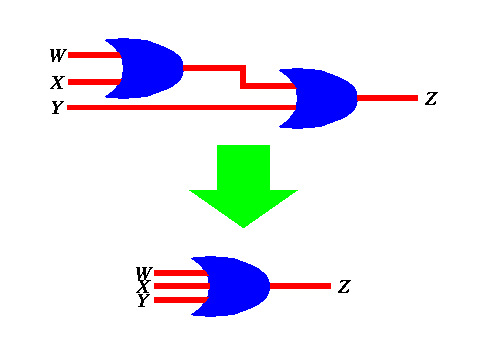The above could be also done for AND gates. For example the figure below shows two different ways of implementing a 4-input AND gate from 2-input AND gates.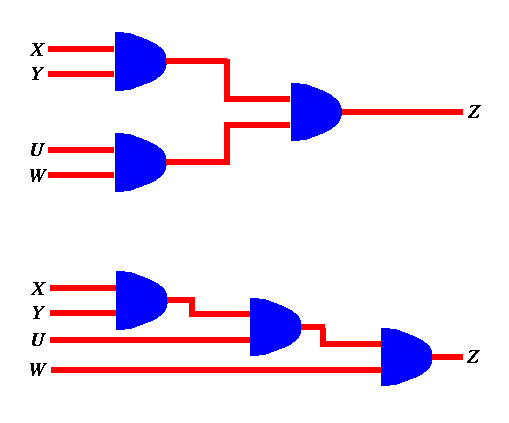# From a circuit to its truth table

The above two examples are simple, since one can predict the behavior of many AND gates connected together or the behavior of many OR gates connected together. Generally speaking, however, this may not be that simple. Given an arbitrary circuit, we can find out what it does by constructing its truth table , which is a tabultaion of the circuit output for all possible input combinations. Take for example the circuit below.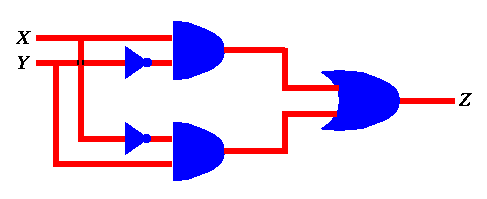First, we notice that it has two inputs, X and Y. Each one of these inputs may assume one of two values 0 and 1. Thus the truth table for that circuit will have four entries. For each such entry we have to find out the output Z. We do so by labeling the circuit. For example, if X = 0 and Y = 0 , we can label the various inputs and outputs of all gates until we find out that Z = 0 as shown below...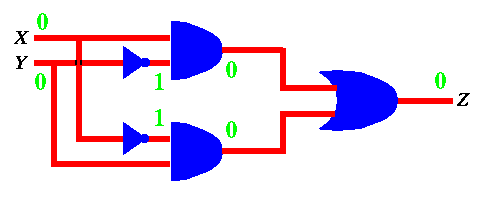Similarily, we can do it for X = 0 and Y = 1 to get Z = 1 as shown below...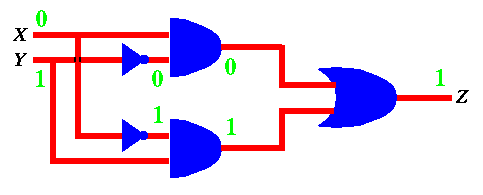If we do this for all 4 input combinations, we get the truth table below.

```  XOR --------------
|  X  Y  |  Z  |
| -------+-----
|  0  0  |  0  |
|  0  1  |  1  |
|  1  0  |  1  |
|  1  1  |  0  |
--------------
```
From this table we notice that the behavior of the circuit is such as to produce an output of 1 if both inputs are not the same. This is called the exclusive or circuit (XOR). It produces a 1 when either one of its inputs is 1 but not both.

The above procedure for finding out what the truth table for a particular circuit is could be repeated for any circuit.

# From a truth table to a circuit

Given the truth table of a particular function, one can build an equivalent logical circuit by following the following 2-step procedure.

First, one has to identify all the "rows" in the truth table for which the output is a 1. Each one of these rows identifies a combination of inputs for which the output must be 1. Thus, one may say that the output must be 1 if the first such combination is present, or the second is present, or the third is present, etc... Thus the output of this circuit is the output of an OR gate whose inputs are the outputs of a number of smaller sub-circuits, each corresponding to a given row in the truth table with an output of 1 .

Second, for each row in the truth table (and thus for every sub-circuit connected to the OR gate), we notice that a particular pattern for the inputs must be present. Such a pattern can be tested using an AND gate with as many inputs as there are inputs to the truth table. A particular input to the AND gate is inverted (using a NOT gate) if its corresponding input in the truth table is 0.

Let's look at an example... Assume we want to build a circuit that has three binary inputs W, X, and Y and one output Z, which is to be 1 when all three inputs are identical (i.e. all 0 or all 1). The tuth table for such a circuit is shown below.

```Equivalence -----------------
|  W  X  Y  |  Z  |
| ----------+-----
|  0  0  0  |  1  |
|  0  0  1  |  0  |
|  0  1  0  |  0  |
|  0  1  1  |  0  |
|  1  0  0  |  0  |
|  1  0  1  |  0  |
|  1  1  0  |  0  |
|  1  1  1  |  1  |
-----------------
```
Following the first step of the procedure outlined above, we conclude that the output of the circuit we have to build is the output of an OR gate with two inputs, corresponding to row 1 and row 8 in the above table (the two rows with Z=1). Following the second step of the procedure outlined above, we conclude that the first input of the OR gate (correpsonding to row 1) comes from an AND gate with three inputs, each of which is inverted using a NOT gate. Similarily, we conclude that the second input of the OR gate (correpsonding to row 8) comes from an AND gate with three inputs, none of which is inverted. Thus the circuit to implement the table above is as shown below.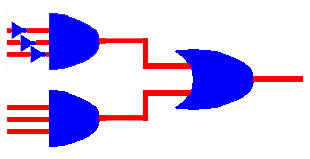In class (and also in the textbook), we learned how to add two binary numbers. Basically, we proceed from the rightmost (least significant) bit position to the leftmost (most significant) bit position. In each position, we add three binary digits X, Y, and Cin and as a result we get two binary digits S and Cout. X and Y are the bits from the two numbers we want to add. Cin is the "carry-in" from the previous bit position, and Cout is the "carry-out" to the next bit position. Let's look at an example...
```   1  1  1  1  0  0  0  0     <--- Cin

0  1  0  1  1  1  0  1     <--- X
+
0  0  1  1  1  0  1  0     <--- Y

------------------------

1  0  0  1  0  1  1  1     <--- S

0  1  1  1  1  0  0  0     <--- Cout
```
Since we use the exact same method in computing the S and Cout bits for all the bit positions, then it is possible to do addition if we could design a circuit that does the addition for one bit position and then get as many of these circuits as there are positions in the numbers we want to add.

In order to design such a above circuit, we have to come up with its truth tables for the two outputs S and Cout. Once we have done that, we can draw the circuit equivalent to these truth tables as shown above.

The truth table for the S as a function of the inputs X and Y and Cin of the circuit is given below.

``` S   -----------------
|  X  Y  Cin|  Z  |
|-----------+-----
|  0  0  0  |  0  |
|  0  0  1  |  1  |
|  0  1  0  |  1  |
|  0  1  1  |  0  |
|  1  0  0  |  1  |
|  1  0  1  |  0  |
|  1  1  0  |  0  |
|  1  1  1  |  1  |
-----------------
```
The truth table for the Cout as a function of the inputs X and Y and Cin of the circuit is given below.
```Cout -----------------
|  X  Y  Cin|  Z  |
|-----------+-----
|  0  0  0  |  0  |
|  0  0  1  |  0  |
|  0  1  0  |  0  |
|  0  1  1  |  1  |
|  1  0  0  |  0  |
|  1  0  1  |  1  |
|  1  1  0  |  1  |
|  1  1  1  |  1  |
-----------------
```
Once the circuits equivalent to the above truth tables are built, we could pack them in a "box" that we would call a one-bit adder as shown below.By connecting several such one-bit adders we could obtain adders that are capable of adding any number of bits we want! For example, a 5-bit adder would look as follows...```Created on: April 13, 1995.